# LTX Units Heat Duty

To calculate the heat duty it must be remembered that the pressure drop through the choke is instantaneous. That is, no heat is absorbed or lost, but there is a temperature change. This is an adiabatic expansion of the gas with no change in enthalpy. Flow through the coils is a constant
pressure process, except for the small amount of pressure drop due to friction. Thus, the change in enthalpy of the gas is equal to the heat absorbed.

The heat duty is best calculated with a process simulation program. This will account for phase changes as the fluid passes through the choke. It will balance the enthalpies and accurately predict the change in
temperature across the choke. Heat duty should be checked for various combinations of inlet temperature, pressure, flow rate, and outlet temperature and pressure, so as to determine the most critical combination.

The heat duty can be approximately calculated using the techniques described in Chapter 2 once the required change in temperature is known. The change in temperature due lo pressure drop through the
choke can be approximated from Figure 4-8, The hydrate temperature can be calculated as described in Chapter 4, and the outlet wellstream temperature selected at approximately 10 °F above the hydrate temperature. The total temperature change for calculating gas, oil and water heat duties is then:The general heat duty for multi-phase streams is expressed as: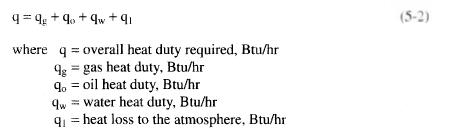The amount of heat required to heat the gas produced from the wellstream is calculated using the following equation: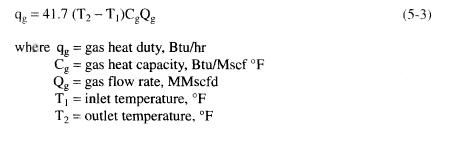The amount of heat required to heat any condensate or oil produced with the gas is calculated using the following equation: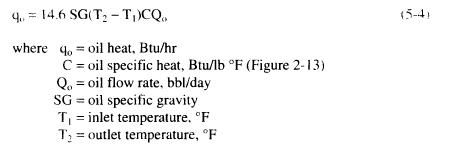The amount of heat required to heat any free water produced with the gas is calculated using the following equation: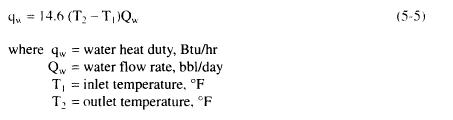Heat loss varies greatly with weather conditions and is usually the greatest in heavy rain and extreme cold. As an approximation it can be assumed that the heat lost from the heater to the atmosphere is less than 10% of the process heat duty. Therefore: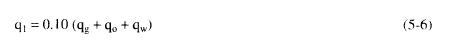The heat duty may have to be checked for various combinations of inlet temperature and pressure, flow rate, and outlet temperature and pressure to determine the most critical combinations.